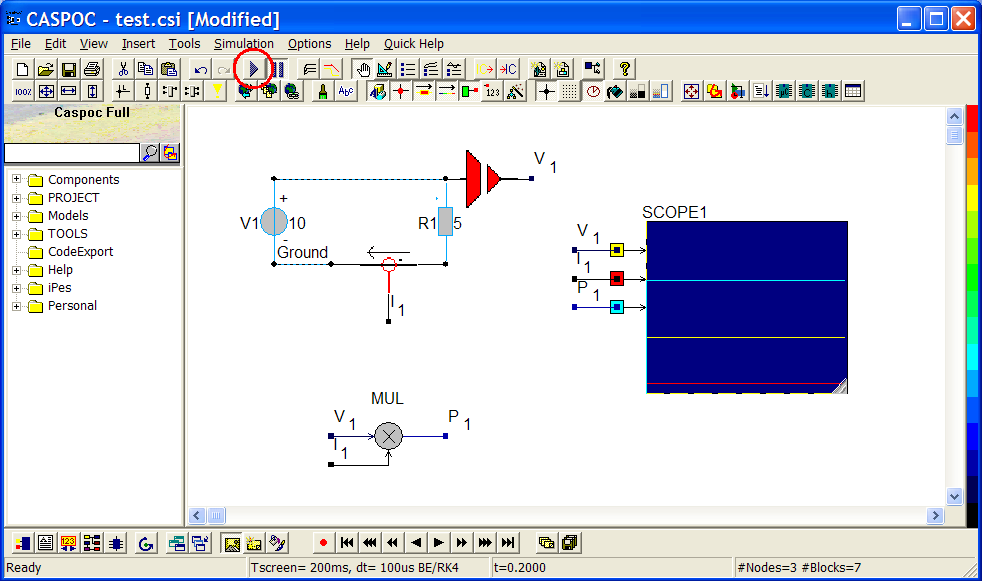## Scope

SCOPE is a very important component in CASPOC to assist users to observe their simulation result easily. The main function of SCOPE is to collect the statistics for your simulation output which can be voltage, current, power, position or any kind of information in your power electrics. During the collection, SCOPE can display the simulation result simultaneously while the simulation is running. Also after the simulation, users can check the simulation result by right-clicking the SCOPE component and modifying the data setting in its menu bar. The simulation result is closely linked to the parameters in your simulation schematic, like time step and simulation time (Tscreen). Users are at liberty to put any SCOPE in any place as they want in order to measure different output data in their power electrics. In the chapter, we will highlight some common functions to help you understand how SCOPE works easily.

### How to get SCOPE component and connect SCOPE to your simulation model: voltage, current

Voltage and current data are the most common information which users often observe during the simulation. If you only need to measure the voltage value on any node or the current value through any component, you can simply add a SCOPE component to reach your goal. Let�s have a look at the following simple example.

Left-click a resistor R and release from Components/Circuit/RLC and then click to put it on the workscreen. Include Reference Label �Ground� or �0�! Automatically Insert? Yes. Right-click the resistor R1 and change the parameter to 5(step 1). Left-click a voltage source V and release from Components/Circuit/Sources and then click to put it on the workscreen. Right-click the voltage source V1 and change the parameter to 10 (step 2). Left-click a SCOPE and release from the left-bottom corner of CASPOC and then click to put it in the right side of the resistor R (step 3).Enlarge the size of SCOPE by using your cursor (step1). The input traces will also increase after the enlargement (step 2)Then we can start to define the input traces for SCOPE1. Connect the first yellow input trace to the node between the resistor R1 and voltage source V1. (Left-click the node and drag to the first yellow input trace. Then release.)Connect the resistor R1 and SCOPE to measure the current value through R1. In CASPOC, there are two simple ways to measure current values without using additional current sensor.

First, left-click the resistor and drag to the second red input trace. Then release! Make sure you can see a dotted line between R1 and the red input trace as below. If not, delete the wire and redo it again.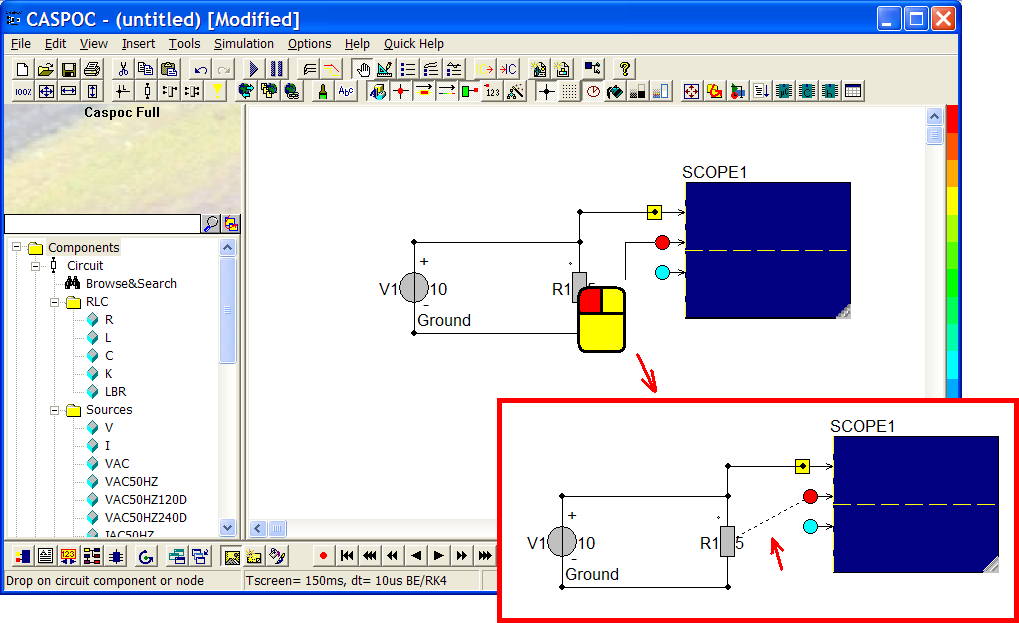The second way is to right-click the second red input trace(step 1). Then launch a popup and enter the value to �Trace=Current through: R1�. Click ok. (step 2). Similarly, Make sure you can see a dotted line between R1 and the red input trace as below (step 3).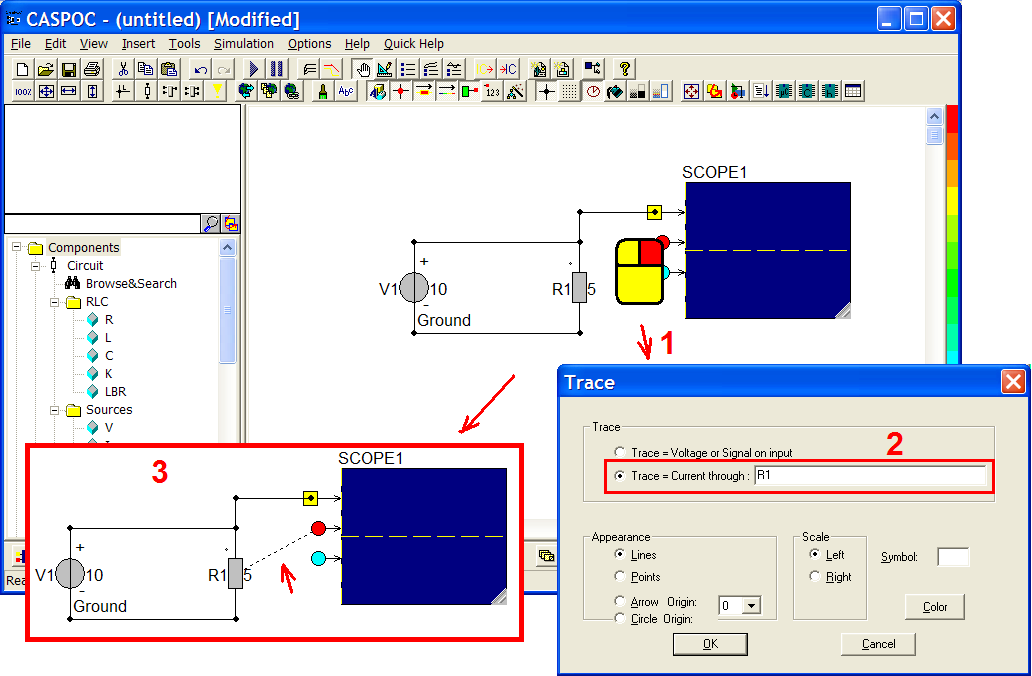### How to open SCOPE and its menu bar

After finishing the setting of SCOPE, click �initializes and starts the simulation� to run the simulation (step 1). Wait the simulation finishes, and then right-click on the SCOPE and open the popup window of SCOPE (step 2).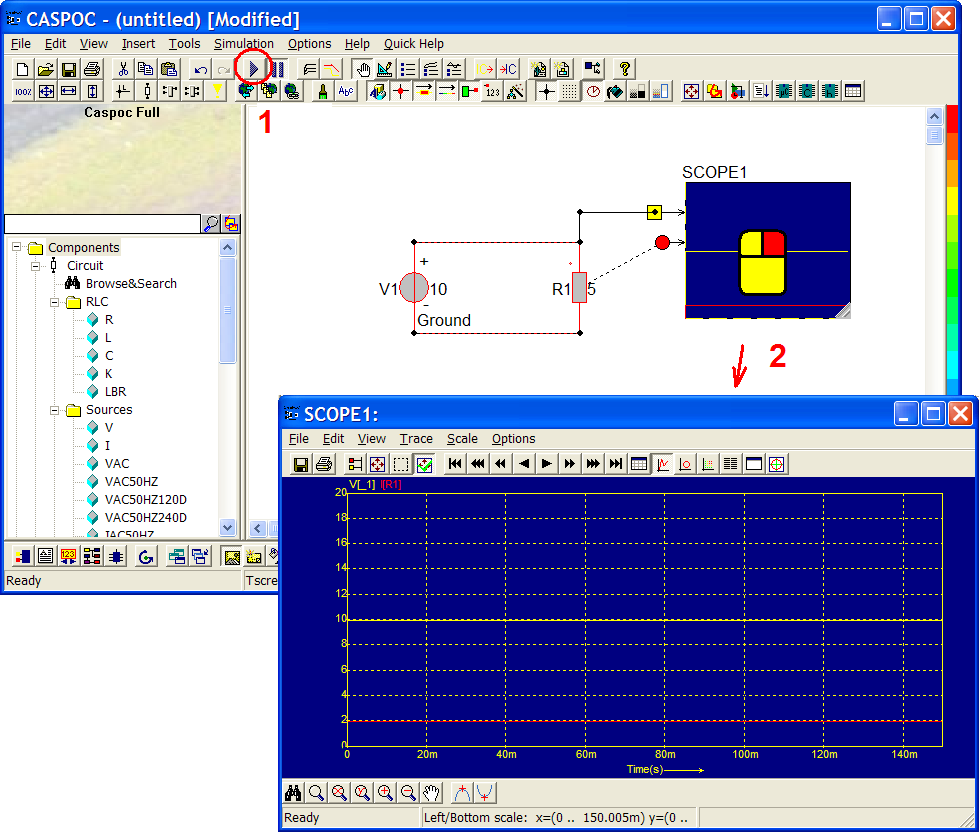Here, we can find the menu bar in the top of the SCOPE window. From left to right as below:

• File- export (.txt, .emf, .eps) or import (.txt, .dat) simulation result.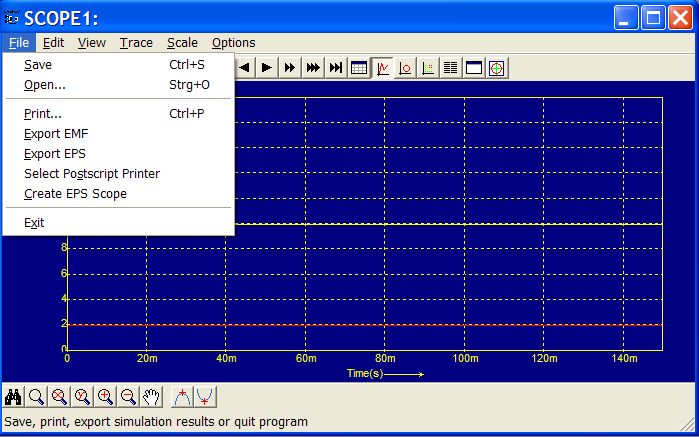• View- change data view in time domain, XY Writer (compare two data rows as X-axis and Y-axis) or DFT (digital Fourier transform).�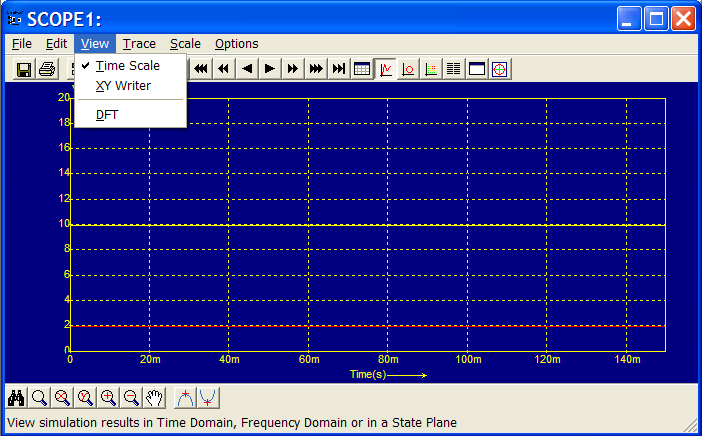• Trace- set the parameters for different traces. Each scope can support 20 traces maximally. In this example, we only use two traces so that available traces are trace 1 and 2.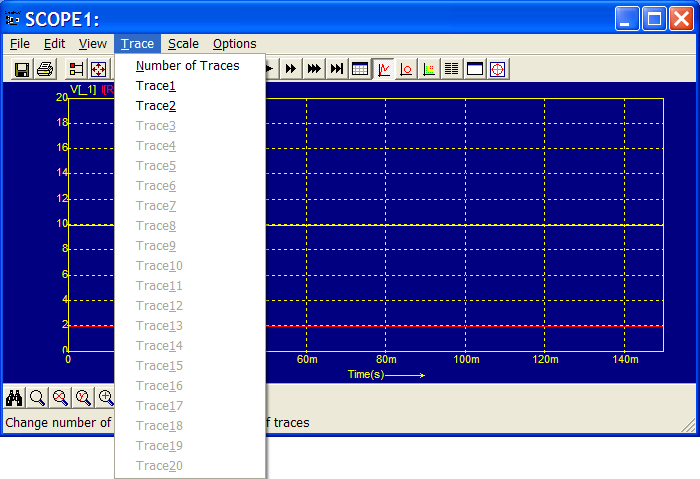• Scale- the scale setting for the current chart. Users can resize, zoom in/out or change the parameters of scale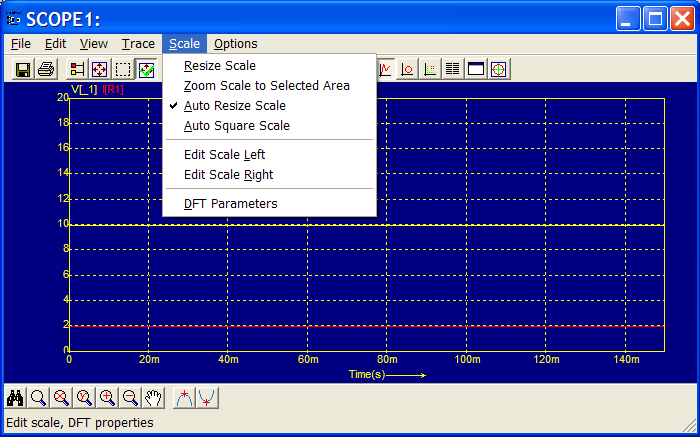• Options- display setting for the chart.### How to save data and simulation result (& how to read the export data)

The data collection can also be saved as a text file. You can click File/Save from the menu bar or click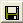�the shortcut button for saving file (step 1). Save the text file in any folder which you want (step 2). Then open the txt file by any text editor (step 3).

Here we named the file as SCOPE1.txt. In the file, we can see there are three columns which stand for simulation time, first input trace and second input trace. In SCOPE, every data column depends on the corresponding input trace. Here the first yellow input trace is the voltage values and the second red input trace is the current values.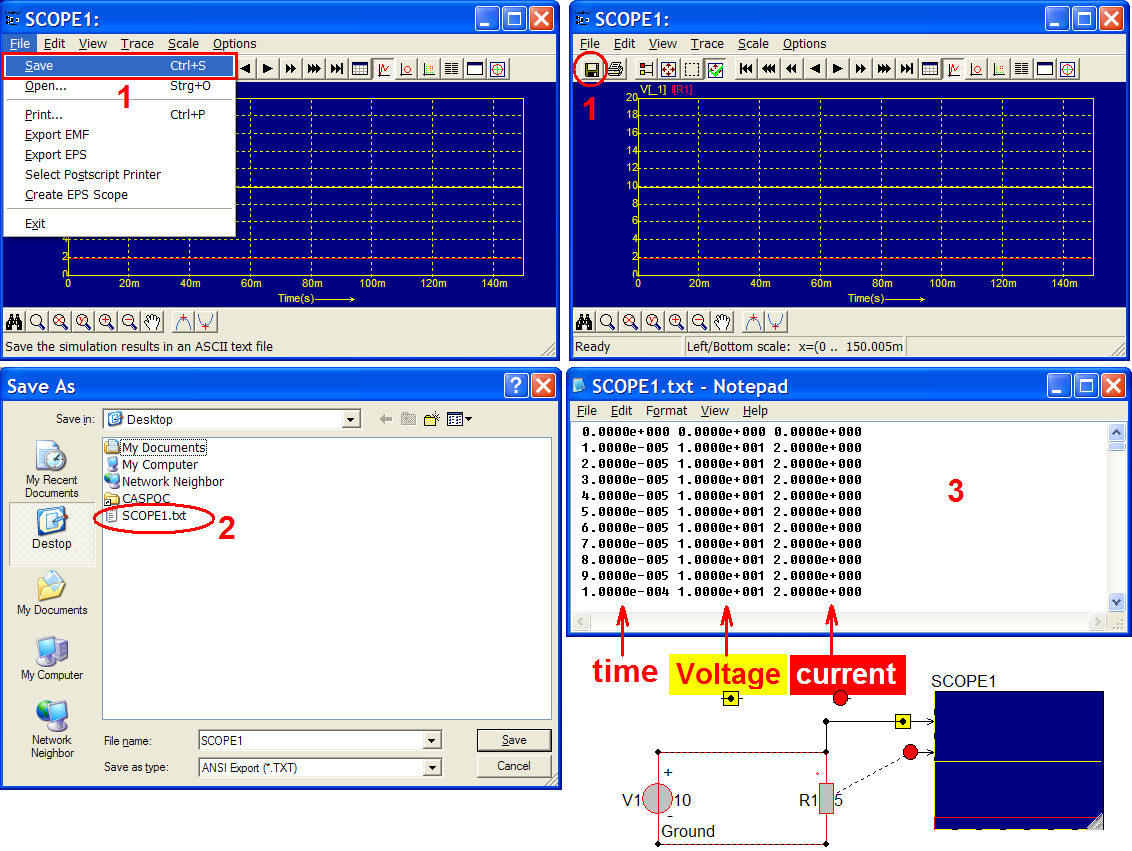All the data are recorded according to the simulation time and step size. In this schematic, simulation time is 150ms (Tscreen) and step size (dt) is 10us. So during the simulation, SCOPE will start to record all the data every 0.00001 second (10us) from 0 to 0.15 seconds (150ms). The simulation time and step size will also be shown on the status bar as below.### How to change the unit of recorded data (step size)

If you want to change the time step or simulation in order to get more detailed or rougher data record, you can go to Simulation/Simulation Parameters in menu bar or click the shortcut button�(step 1) and modify the parameters as below. In this case, we change the simulation time (Tscreen) to 200ms (0.2 seconds) and the step size (dt) to 100us (0.0001 seconds) (step 2). Then click ok and we can see the status bar shows Tscreen=200ms and dt=100us (step 3).Re-execute the simulation by clicking �Initializes and starts the simulation�. After the simulation, right-click the SCOPE and save the simulation result as below. �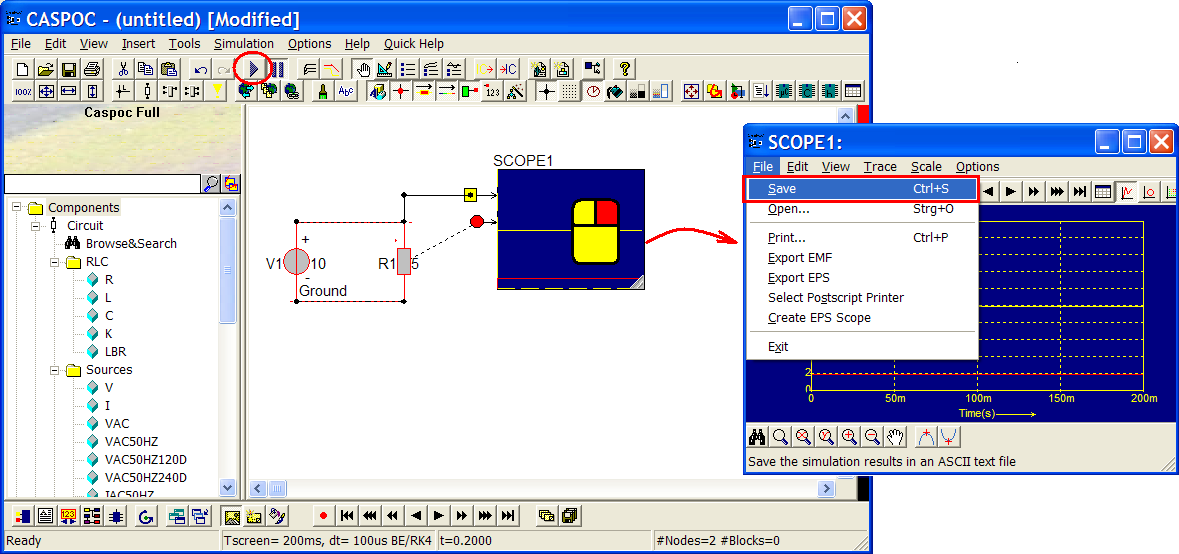Open the text file which you just saved, and compare to the simulation parameters you set. From the file, we can find SCOPE records data every 0.0001 seconds (100us) from 0 to 0.2 seconds (200ms).### Data display: Time scale, XY Writer, Trace appearance

In the above description, all the data are recorded in time domain. And we can change the display mode according to the properties of the data. There are some simple options, like XY-Writer and DFT.

XY-Writer is a very common and useful option in power electrics simulation. For example, if users want to observe the V-I character curve, we can set voltage and current values as X-axis and Y-axis data respectively. In SCOPE, while you choose XY-Writer, the odd input traces (like trace 1, 3, 5 �) will be the X-axis data and the even input traces (like trace 2, 4, 6�) will be the Y-axis data. In terms of that, when you want to set up a XY-Writer SCOPE, the input trace numbers have to be even. And all the odd and even input traces have better to be the same characters.

In the following sample, we add one more resistor R2 and voltage source V2. Then change R2 to 2 and V2 to 10. There is a ground connection in the node between R2 and V2. Connect the node in the upper end of R2 to the third blue input trace and connect the fourth green input trace to the resistor R2 as below. Then re-execute the simulation by clicking �Initializes and starts the simulation� and right-click the SCOPE to open the simulation result.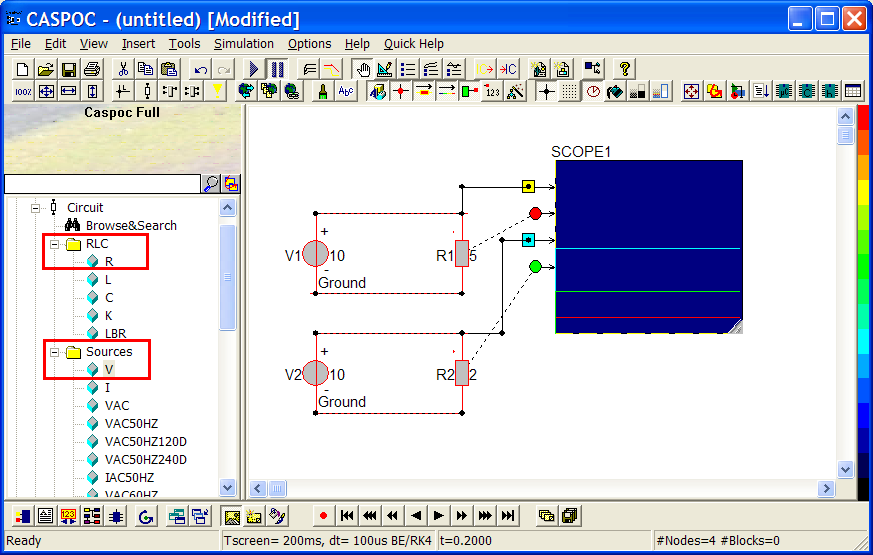Select View/XY Writer in the menu bar of SCOPE1 (step 1). Then the original simulation result changes from time domain to XY-Writer as below. In this sample, the first and third input traces are the voltage values of the two circuits (step 2); the second and the fourth input traces are the current values of the two circuits (step 3). So before change to XY-Writer, the X-axis is simulation time. After changing to XY-Writer, the data display will use the two voltage data as X-axis and the two current data as Y-axis.

On the workscreen, we can also see on the first and second input traces are drawn a frame round, as well as the third and fourth traces (step 4).�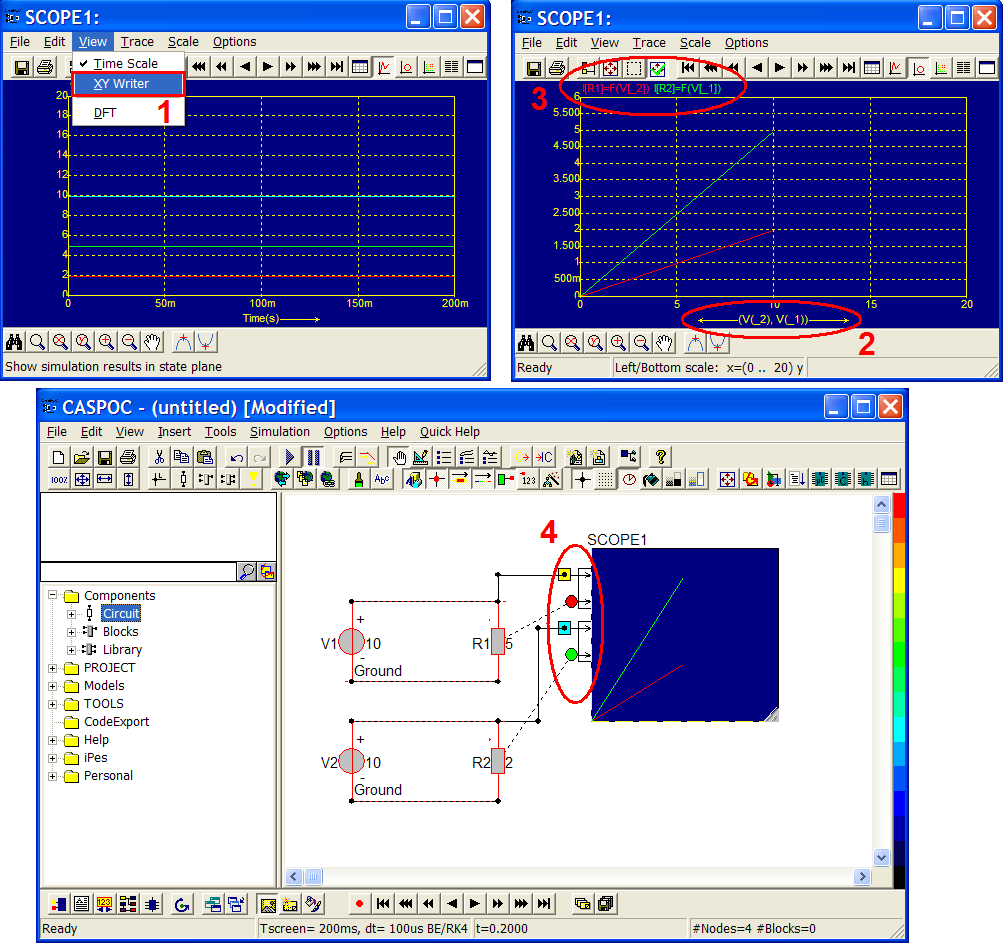Users can set the parameters on all the different traces. All the traces will follow the colorful input dots in the left side of SCOPE. Here we can have a simple test on trace 1. Trace 1 is the first input trace of SCOPE with yellow dot. Go to Trace/Trace 1 and then get a popup window as below. Because trace 1 is the voltage data, we can see the trace = voltage at input ( ) on the popup. If the trace is current data, the popup will show the trace = current through: (component name). Otherwise, popup will show the trace = signal at input ( ) if we use some sensors, like speed, position, force and so on. We will show a sample later in this tutorial.

On the right side of popup, we can give a symbol to mark the curve on SCOPE. Here we type V_1 then we can find V_1 on SCOPE. In principle, V_1 stands for the first yellow input trace 1. But the third blue input trace 3 overlays trace 1. That�s the grounds that we cannot see the yellow curve.In the scale setting, users can set up the scale side for all the traces in your SCOPE (step 1). For all the traces, the default scale is in left side with yellow color. If we choose any trace and change its scale to right side, we will see there is another new scale in right side with purple color as below. Then the traces which use the left scale will be drawn a yellow frame round; the traces which use the right scale will be drawn a purple frame round (step 2).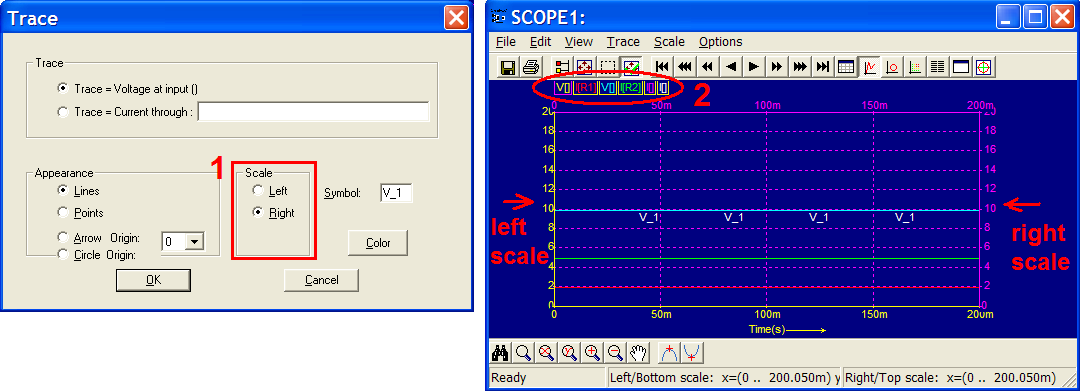Go back to the workscreen and enlarge SCOPE1 in order to get more input traces. Left-click the node between R2 and V2 and drag to the fifth pink input trace of SCOPE1 then release (step 1). Right-click the sixth white input trace of SCOPE1 (step 2) and enter Trace=Current Through: R2. Then change the appearance from �line� to �Arrow Origin 0� (step 3). The signal inputs of fifth and sixth traces are the same as third and fourth ones, and the only difference is the appearance. Our goal here is to configure an arrow which will show the current data point of fourth trace. No matter arrow or circle, the two appearances are used to show the current data point. Users just need to select the origin is from the available list. In this case, we have the origin, trace 2 and trace 4. The available traces depend on the input traces of the SCOPE as below.### How to use sensors (measure data)

From the above, we only see how to measure the voltage and current values by SCOPE. But in power electrics, there are still a lot of information we need, like power, harmonics and other mechanical data (speed, position, etc...).

CASPOC provides users many sensors which can be used in their simulation schematics. You can find all the sensors in the directory Components/Library/Sensor. (Note: there are also some sensors or probes in other directories according to their properties.)

Here we keep the voltage source V1 and the resistor R1. The parameter of V1 is 10 volt and R1 is 5 ohm. Put one SCOPE component and enlarge it as below. Note: delete the lower connection between R1 and V1.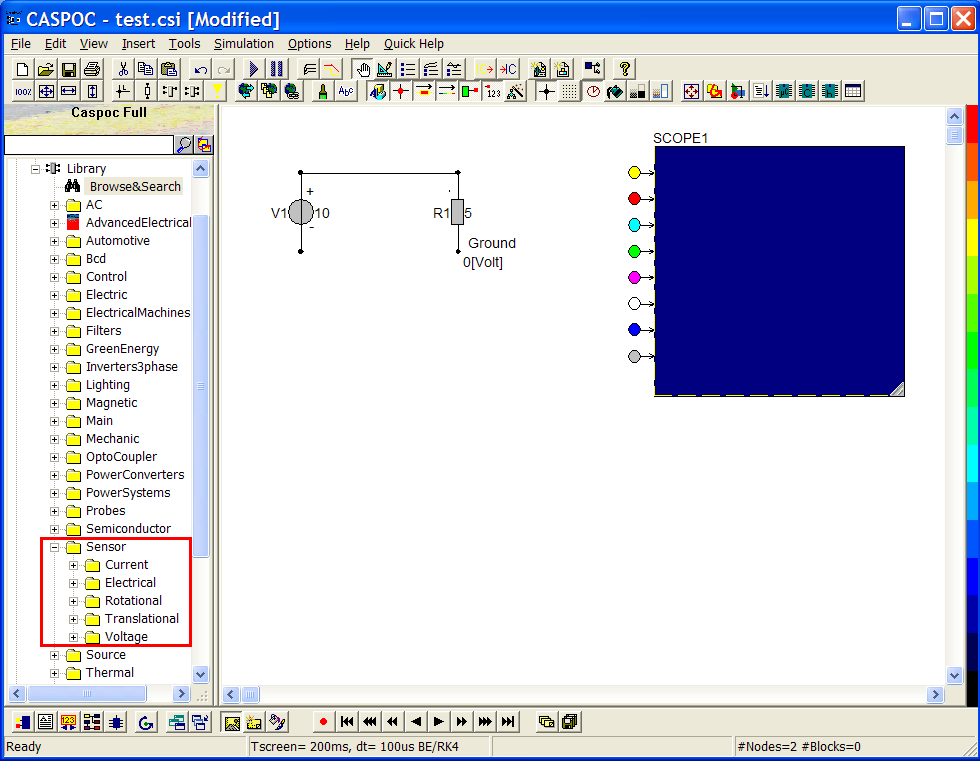Left-click the current sensor (located in Components/Library/Sensor/Current/i) and release your mouse button. Move your cursor to the workscreen and left-click. Do the same steps with the voltage sensor (located in Components/Library/Sensor/Voltage/v).

Then we can see on the sensors there are some different round dots and square dots. In tutorials, we already explained the round dots transmit current and can only connect to circuits; square dots transmit signals and can only connect to mechanical components, mathematical operation, and SCOPE. On the other hand, round dots can only connect to other round dots, as well as square dots.��Then left-click the current and voltage sensors NOT release and drag them to connect the schematic as the following configuration. Notice the round dots of the sensors connect to the round dots of the circuit too. Finally connect the left side of current sensor with the lower side of voltage source (step 1).

After finishing the connection of round dots, left-click the square dot of voltage sensor not release and drag the cursor to the first input trace of SCOPE1. Then left-click the square dot of current sensor not release and drag the cursor to the second input trace of SCOPE1 (step 2).

Execute the simulation by clicking �Initializes and Starts the simulation� (step 3).��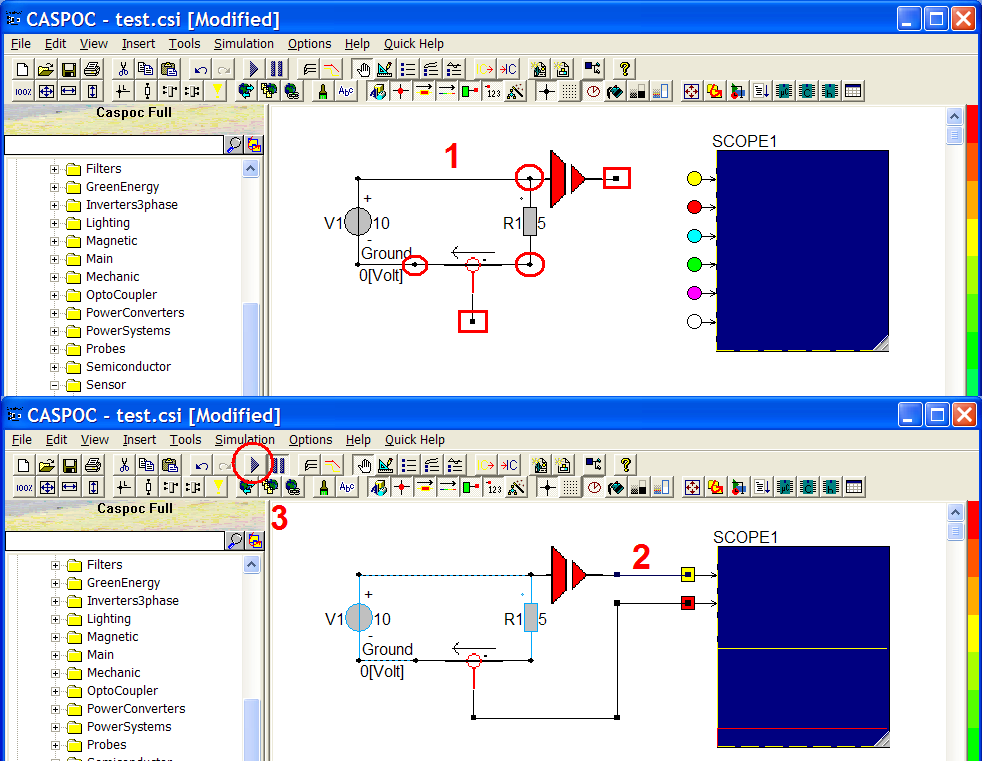Sometimes we don�t want to drag wire between sensors and SCOPE because of considering the tidy appearance of the schematic. Here we can choose the other way to connect the square dots and SCOPE.

Right-click the square dot of the voltage sensor and name this label V_1 (step 1). Similarly, right-click the square dot of the current sensor and name this label I_1 (step 2).Use your cursor left-click and NOT release the first yellow input trace. Drag to the left side a bit to have a square dot in the left of the yellow dot.�Then right-click the square dot (step 1) and name the label V_1 (step 2) in the popup.�Do the same steps in the second input trace. Right-click the square dot in the left side of the second red dot (step 1) and name this label I_1 (step 2).Then we can find there are two square dots with the same name V_1 and the other two dots with the same name I_1. In CASPOC, all the nodes with the same name will be supposed to be connected together even there is no physical wire. Click �Initializes and Starts the simulation� to run this simulation. You will find the result is the same as the previous one with physical wires.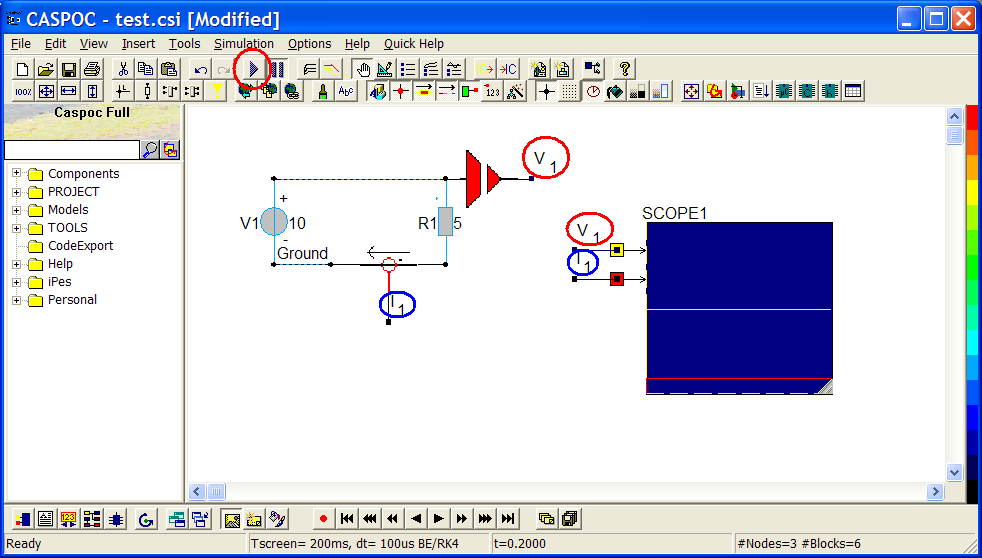### How to get the calculated data (using blocks) and show the result in SCOPE

Besides the measure values from the power electrics, users can also add some mathematical operator and send the result to SCOPE for display or save as a text file. Here we use the multination operator to multiple V_1 and I_1 in order to get the power value of the schematic.

Left-click the �MUL� (located in Components/Blocks/Math) and release your mouse button. Then left-click on the workscreen to put the �MUL� operator as below. Notice that here we can see there are three square dots in the MUL operator. That�s because MUL is not a component for circuit to transmit current.Right-click the first square dot in the left side of MUL and name this label V_1.Right-click the second square dot in the left side of MUL and name this label I_1.Right-click the square dot in the right side of MUL and name this label P_1.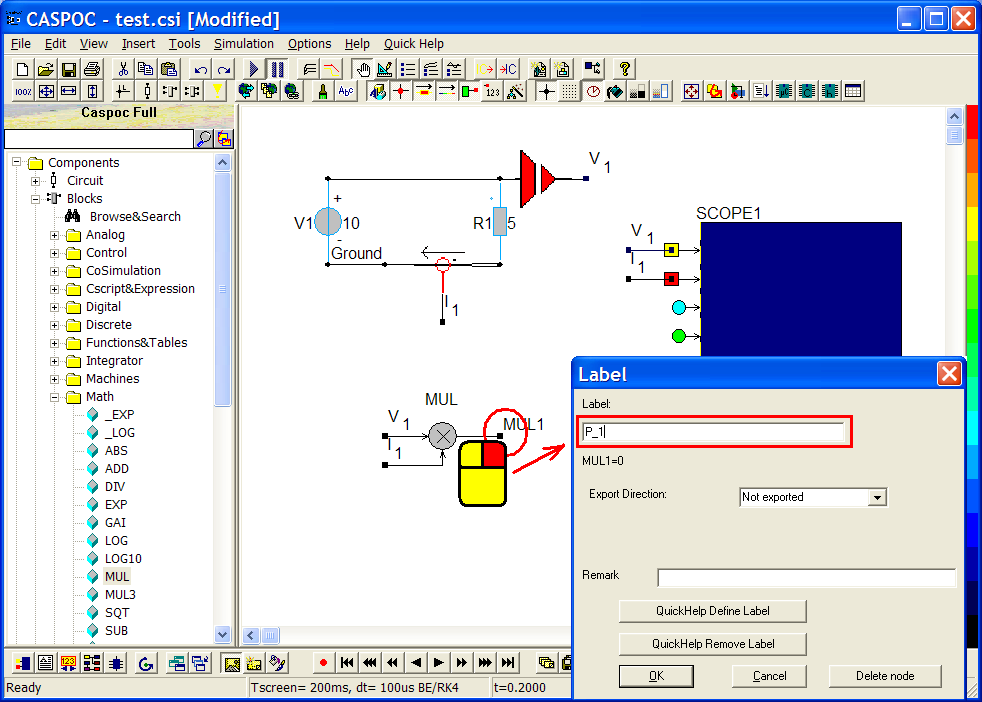Similarly, use your cursor left-click and NOT release the third blue input trace. Drag to the left side a bit to have a square dot in the left of the blue dot. Then right-click the square dot and name the label P_1 to receive the calculation output from P_1 in MUL operator.�Make sure the schematic has the right label V_1, I_1 and P_1 as below. Then execute the simulation by clicking �Initializes and Starts the simulation�. The blue line (trace 3) on SCOPE1 is the calculation power from voltage and current sensor. �